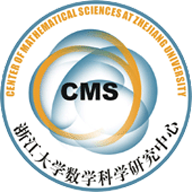Pinching theorems for self-shrinkers of higher codimensions

In this paper, we investigate the pinching phenomena of the tracefree second fundamental form of complete self-shrinkers of higher codimension. Firstly, assuming the mean curvature is nonzero everywhere and the self-shrinker is of polynomial volume growth, we prove that if the tracefree second fundamental form $\mathring{A}$ satisfies $||\mathring{A}||_{n}<C(n)$ for a positive constant $C(n)$ depending only on the dimension $n$ of the self-shrinker, then it is isometric to the sphere $\mathbb{S}^{n}(\sqrt{2n})$. Secondly, we show if the mean curvature vector $H$ of the self-shrinker satisfies $\sup|H|<\sqrt{\frac{n}{2}}$ and $\mathring{A}$ satisfies $||\mathring{A}||_{n}<D(n,\sup|H|)$ for a positive constant $D(n,\sup|H|)$ depending $n$ and $\sup|H|$, then it is isometric to the Euclidean space $\mathbb{R}^{n}$. We also obtain some rigidity theorems for self-shrinkers satisfying pointwise curvature pinching conditions on $|\mathring{A}|^{2}$.Pinching theorems for self-shrinkers of higher codimensions.pdf